## Sunday, 15 January 2023

### Happy 2023!Monthly visitors 2021-22. Page views ~3 each.

My main interests in the past year have not been maths and relativity  and I have not posted here for over a year. However, the website remains fairly popular and occasionally attracts useful and nice comments especially from Petra Axolotl who was my very first mentor back in 2018. So I will continue to pay a tenner a year to keep the website going.

Happy 2023, year of the rabbit.

## Monday, 10 January 2022

### New Scientist Christmas Puzzle 2021

The grid contains 46 x 72 = 3,312 letters. Hidden inside it are the 118 elements in the periodic table, 17 known fundamental particles and 20 amino acids that make up proteins. They are revealed here. TAU and TIN occur about 15 and 31 times respectively. Two TINs come out of row 44, column 30, one going up and left, the other up and right. IRON and MUON occur three times. All other multiple occurrences are necessary if one is to have words such as ERBIUM, TERBIUM, YTTERBIUM. MUON should occur twice, the second time in MUONNEUTRINO.

The original puzzle is here. The spreadsheet and more are here.

## Tuesday, 7 December 2021

### Powers of sine and cosine as multiple angles

I had a problem which required integrating powers of sines and cosines.
I used integral-calculator to do the work up to a power of six. Its results are shown below. $$\fbox{\begin{matrix}n&\int{\cos^n{\theta}d\theta}&\int{\sin^n{\theta}d\theta}\\2&\frac{\sin{\left(2\theta\right)}+2\theta}{4}&\frac{-\sin{\left(2\theta\right)}+2\theta}{4}\\3&\sin{\theta}-\frac{\sin^3{\theta}}{3}&\frac{\cos^3{\theta}}{3}-\cos{\theta}\\4&\frac{\sin{\left(4\theta\right)}+8\sin{\left(2\theta\right)}+12\theta}{32}&\frac{\sin{\left(4\theta\right)}-8\sin{\left(2\theta\right)}+12\theta}{32}\\5&\frac{\sin^5{\theta}}{5}-\frac{2\sin^3{\theta}}{3}+\sin{\theta}&-\frac{\cos^5{\theta}}{5}+\frac{2\cos^3{\theta}}{3}-\cos{\theta}\\6&\frac{\sin{\left(6\theta\right)}+9\sin{\left(4\theta\right)}+45\sin{\left(2\theta\right)}+60\theta}{192}&-\frac{\sin{\left(6\theta\right)}-9\sin{\left(4\theta\right)}+45\sin{\left(2\theta\right)}-60\theta}{192}\\\end{matrix}}$$
There appears to be a pattern. But at the moment it is veiled. The integrals of odd powers can be found by a crafty substitution which I found here. It gives$$\int\sin^n{\theta}d\theta=\sum_{l=0}^{l=\frac{n-1}{2}}{\left(-1\right)^{l+1}B_l^{\frac{n-1}{2}}}\frac{\cos^{2l+1}{\theta}}{2l+1}+C,\ for\ odd\ n$$$$\int\cos^n{\theta}d\theta=\sum_{l=0}^{l=\frac{n-1}{2}}{\left(-1\right)^lB_l^{\frac{n-1}{2}}\frac{\sin^{2l+1}{\theta}}{2l+1}}+C,\ for\ odd\ n$$where $B_l^n$ is the binomial coefficient$$B_l^n=\frac{n!}{l!\left(n-l\right)!}$$The integrals of even powers are not so simple. I ventured into multiple angle expressions for $\cos^n{\theta},\sin^n{\theta}$ using the Chebyshev method. Multiple angles are easy integrate. I ended up with the Christmas trees at the top.

If you're interested read it here: Sine and Cosine formulas.pdf (12 pages).

## Monday, 22 November 2021

### 3D-grapher at math3d.org

I was practicing my tensor analysis and calculated a normal vector to a surface. I wanted to see it looked orthogonal to the surface. It didn't really. So I made a tangent plane to the surface. The normal vector still looks a bit un-orthogonal but it passes all the mathematical tests.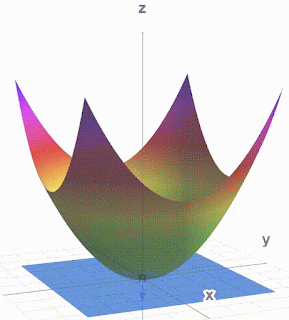Paraboloid, Normal vector and tangent plane $\phi_2\left(\vec{x}\right)=\left(x^1\right)^2+\left(x^2\right)^2-x^3=0$

You can play with this at and stop it from moving.
I also used Debut Video Capture to make a .avi from math3d and then cloudconvert.com to make the .gif. It is simpler than ezgif.com.

Other surfaces and vectors: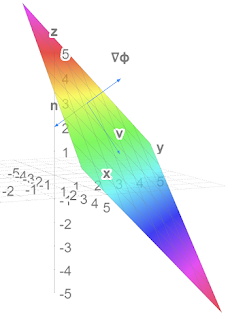Plane and normal vectors (not v)Play at https://www.math3d.org/fq5CtGqYa$\phi_1\left(\vec{x}\right)=x^1+x^2+x^3=5$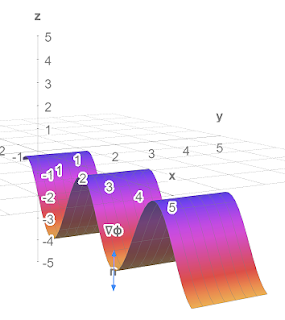Sine wave with normal vectors Play at https://www.math3d.org/D8xf0gZMT$\phi_3\left(\vec{x}\right)=x^3+r_0\cos{\left(kx^1\right)}=-4$

Lots more tools on Physics Forums at

## Monday, 25 October 2021

### Cubic equations and elliptic curves

At what $c$ does the curve change from one type to the other and what then is the leftmost point of the curve?

Answers$$c=\sqrt{\frac{32}{27}}\ \ ,\ \ x=-\sqrt{\frac{8}{3}}$$Here's why PF Challenge 2110#14.pdf (7 pages).

## Tuesday, 12 October 2021

### The Parallel Propagator and using it on the surface of a sphere

The three page Appendix I is on the parallel propagator which gives a general solution to the parallel transport equation of a vector. The appendix shows how to get this from the parallel transport equation which is, for a vector $V^\nu$ transported along a curve $x^\mu\left(\lambda\right)$ $$\frac{dx^\mu}{d\lambda}\partial_\mu V^\nu+\frac{dx^\mu}{d\lambda}\Gamma_{\mu\sigma}^\nu V^\sigma=0$$First we note that the transported vector can be calculated by$$V^\mu\left(\lambda\right)=P_{\ \ \rho}^\mu\left(\lambda,\lambda_0\right)V^\rho\left(\lambda_0\right)$$where $V^\rho\left(\lambda_0\right)$ is the vector at the start point $x^\mu\left(\lambda_0\right)$ and $P_{\ \ \rho}^\mu\left(\lambda,\lambda_0\right)$ is some matrix which is called the parallel propagator. Next we define another matrix $$A_{\ \ \rho}^\mu\left(\lambda\right)=-\Gamma_{\sigma\rho}^\mu\frac{dx^\sigma}{d\lambda}$$and then show that (dropping indices on the matrices)$$P\left(\lambda,\lambda_0\right)=I+\sum_{n=1}^{n=\infty}T_n$$where $I$ is the identity matrix and $$T_n=\int_{\lambda_0}^{\lambda}{\int_{\lambda_0}^{\eta_n}\int_{\lambda_0}^{\eta_{n-1}}{\ldots\int_{\lambda_0}^{\eta_2}A\left(\eta_n\right)A\left(\eta_{n-1}\right)\ldots A\left(\eta_1\right)}d^n\eta}$$$$=\frac{1}{n!}\int_{\lambda_0}^{\lambda}{\int_{\lambda_0}^{\lambda}\int_{\lambda_0}^{\lambda}{\ldots\int_{\lambda_0}^{\lambda}\mathcal{P}\left[A\left(\eta_n\right)A\left(\eta_{n-1}\right)\ldots A\left(\eta_1\right)\right]}d^n\eta}$$where $\mathcal{P}$ orders the matrices $A\left(\eta_i\right)$ so that $\eta_n\geq\eta_{n-1}\geq\ldots\geq\eta_1$.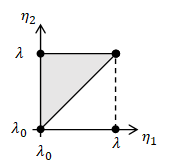2-simplex

The first integral is over $n$-dimensional equilateral right triangles, or $n$-simplices and is quite hard to calculate but $n!$ $n$-simplices make an $n$-cube which makes the second integral which is much easier to calculate. I had a bit of trouble getting my head round all that and I tested it on a few examples including vectors transported along lines of constant latitude.  It all works!

### Vectors transported round latitudes calculated by parallel propagator

Note vector length never changes. $T_n$ was evaluated to 5 decimal places.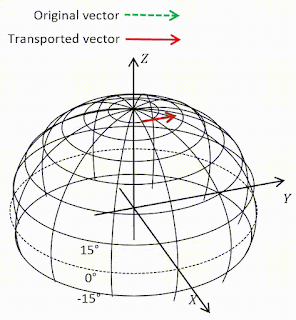Transport at 80°N. Vector barely changes because it's nearly flat up there.$T_n=0$ at $n=25$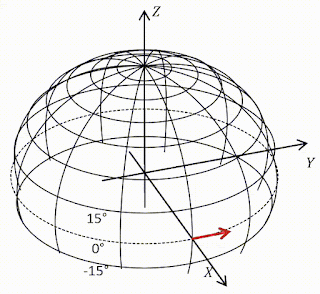Transport round equator. Vector remains parallel because equator is geodesic.$T_n=0$ at $n=1$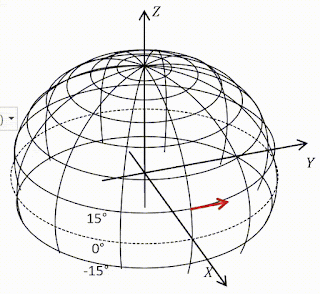Transport at 15°N. Vector rotates down by 87°.$T_n=0$ at $n=11$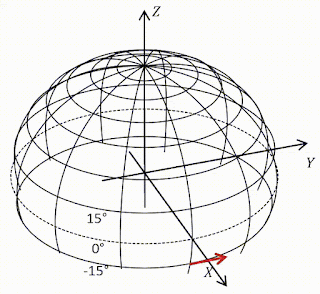Transport at 15°S.Vector rotates up by 87°.$T_n=0$ at $n=11$

## Tuesday, 14 September 2021

### Question

Take the mathematician's view that tangent vectors and directional derivatives are the same thing, $u\equiv\partial_u$. Let $u,v$ be two vector fields and define their commutator in the manner familiar from quantum mechanics [not familiar to me!]$$\left[u,v\right]\equiv\left[\partial_u,\partial_v\right]\equiv\partial_u\partial_v-\partial_v\partial_u$$(a) Derive the following expression for $\left[u,v\right]$ valid in any coordinate basis$$\left[u,v\right]=\left(u^\beta v_{\ \ ,\beta}^\alpha-v^\beta u_{\ \ ,\beta}^\alpha\right)e_\alpha$$Thus despite that it looks like a second-order differential operator, $\left[u,v\right]$ is actually of first order - i.e. it is a tangent vector.
(b) For any basis $\left\{e_\alpha\right\}$ one defines the "commutation coefficients" $c_{\beta\gamma}^{\ \ \ \ \ \alpha}$ and $c_{\beta\gamma\alpha}$ by $$\left[e_\beta,e_\gamma\right]\equiv c_{\beta\gamma}^{\ \ \ \ \ \alpha}e_\alpha;\ \ c_{\beta\gamma\alpha}=g_{\alpha\mu}c_{\beta\gamma}^{\ \ \ \ \ \mu}$$Show that $c_{\beta\gamma}^{\ \ \ \ \ \alpha}=c_{\beta\gamma\alpha}=0$ got any coordinate basis.
(c) Calculate $c_{\hat{\beta}\hat{\gamma}}^{\ \ \ \ \ \hat{\alpha}}$ for the spherical noncoordinate basis of exercise 8.1.